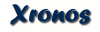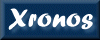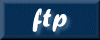```LCMATH (Aug96) xanadu.xronos LCMATH (Aug96) NAME lcmath - Subtracts or adds two binned lightcurves. USAGE lcmath infile bgfile outfile multi multb DESCRIPTION This program takes as input two binned lightcurves and calculates a result lightcurve either subtracting (second from the first) or adding the two input files. The two input files should cover the same time interval. In particular the start time and the stop time of the first lightcurve should be included within the start and stop time of the second lightcurve. Error are prompt if the start and/or the stop are outside of the time interval of the second file. The time resolution of the two files can be different, but the integration time of the first input file should be always less than or equal to that in the seconf input file. The task does not work for input files containing "EVENT" table. Constant scaling and additive factors can be separately input for both lightcurves. By default, the task will apply any vignetting (or collimator) and deadtime correction information contained in the input file (header keywords VIGNET and DEADC) to the result lightcurve. The user may cancel this correction setting the parameter "docor". The columns names recognized by the task are TIME, COUNT, RATE and ERROR. The units (TUNITn keyword) are expected to be 'count' for column name 'COUNT' and 'count/s' for column name RATE. The input file structure is a FITS bintable with data stored in the column COUNT or RATE either as a single element or a vector of element in each row. For the last case the task works correctly only if the column n contains a vector of counts in different channel (the value of the column keyword 1CTYPn should be 'CHANNEL'). The paramaters "emin" and "emax" are applicable in this case. The structure of the output file will have always data stored as a single element in each row. ERRORS If scaling and additive factors are input for both file1 and file2 the derived counts are calculated as: count1=M1*x1 + C1 count2=M2*x2 + C2 where M1 and M2 are the input scaling values for the first and second lightcurve respectively; C1 and C2 are the additive input factors in counts (rescaling for the fractional exposure) and x1 and x2 are the value always in counts read from the files (if the file contains rate x1 and x2 are the value after rescaling for the integration and exposure time). The final results in the output file will be: count3= count1 -/+ count2 divided for the integration time. The error for count3 is calculated as: err3= sqrt( err1**2 + err2**2) where err3, err2, and err1 are the error values for the count3, count2 and count1 respectively. To calculate the individual errors (i.e. err1 for the quantity count1 and err2 for the quantity count2) the user can choose one of the 6 different methods listed below. Method 1 err1=sqrt((sqrt(M1*sx1*sx1))**2 + C1) Method 2 err1=sqrt((M1*sx1)**2) Method 3 err1=sqrt( (sqrt(M1*sx1*sx1))**2) Method 4 err1=sqrt( (M1*sx1)**2 + C1) Method 5 err1=sqrt(count1) Method 6 The error in the output file is set equal to the value of the error of first input file (i.e. err3=sx1) If the file contains an error column, sx1 is the value in the file rescaled for the integration time and exposure, otherwise sx1 is set as the sqrt(x1) or sqrt(x2). If x1 or x2 are 0 the error (sx1 or sx2) is set to 1. If the scaling factor is 1 and the additive factor is 0, the methods 1,2,3,4 are equivalent. PARAMETERS infile [filename] The name of the first input lightcurve. If the task is used in subtraction mode the file should contain source+background data. bgfile [filename] The name of the second input lightcurve. If the task is used in subtraction mode the file should contain background data. outfile [filename] The name of the output result lightcurve. If lcmath is used in the subtracting mode the file contain source data only and the keyword BACKAPP is added or updated. multi = 1. [real] Scaling factor for first input lightcurve. multb = 1. [real] Scaling factor for second input lightcurve (or background lightcurve). (addsubr = no) [boolean] Flag to Add input lightcurve instead of subtract. The default value is set to subtract (addsubr=no no add) the lightcurve. (addi = 0.) [real] Additive offset for first input lightcurve. This value should be given as counts (internally the value corrected for the bin fractional exposure). (addb = 0.) [real] Additive offset for second input lightcurve (or background lightcurve). This value should be given as counts (internally the value is corrected for the bin fractional exposure). (docor = yes) [boolean] Whether to apply the corrections to the result lightcurve. If the input lightcurves have stored in the header the vignetting or collimator (VIGNET keyword) and the deadtime (keyword DEADC) correction, this is also apply by default to the output lightcurve. (emin = 0) [integer] Minimum energy channel to use. Enter 0 for no lower bound. (emax = 0) [integer] Maximum energy channel to use. Enter 0 for no upper bound. (err_mode = 0) [integer] Method to calculate the errors on the individual input lightcurve. The value of the paramater ranges from 1 to 5 (see ERRORS section) and is default to 1. ERROR (tchat = 10) [integer] Terminal chattiness level. (lchat = 0) [integer] Logfile chattiness level. EXAMPLES BUGS Report problems to angelini@lheavx.gsfc.nasa.gov, xanprob@athena.gsfc.nasa.gov. Provide a detailed description of the problem (possible a log file). SEE ALSO ```Please send reports of errors to : xanprob@athena.gsfc.nasa.gov HEASARC Home | Observatories | Archive | Calibration | Software | Tools | Students/Teachers/Public Last modified: Wednesday, 04-Jun-2003 11:35:12 EDT HEASARC support for unencypted FTP access will be ending possibly as early as July 31, 2019. Please see this notice for details.